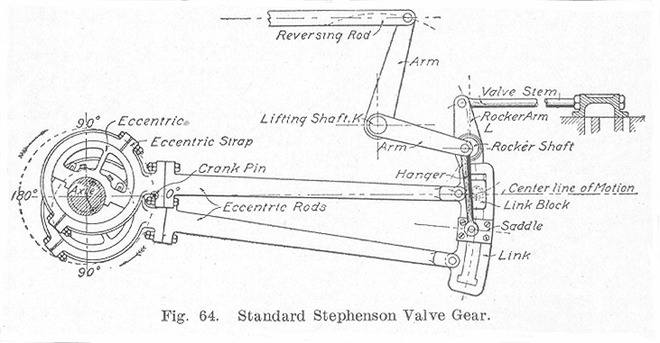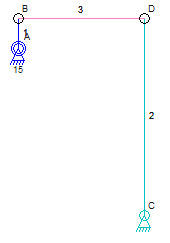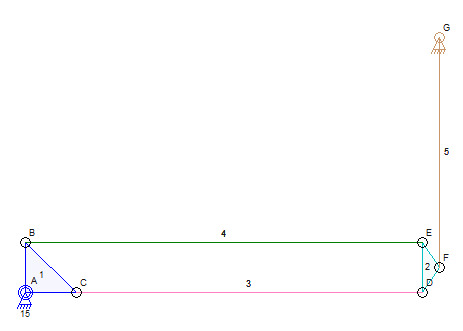Upon further inspection, A Stephenson value gear mechanism cannot be built with the Linkage program. I don’t have the necessary simulation code to handle the complexity of the links.The problem comes from the connection of the “Link” to the rest of the mechanism. The position of that link, the one that contains the slider path, cannot be computed using simple circle-to-circle intersection calculations or circle-to-line calculations.

This problem has always been there for my simulator. An explanation of why this is a problem is in order.

### The Simulator

The simulator code for non-sliding connectors is super simple. Start with a mechanism:The Simulator code will examine the mechanism and find every pair of links that are connected to each other with a not-fixed-in-place connector but also with each having a fixed-in-place connector. Visualize that links 2 and 3 are connected together and each has a fixed connector (B and C). Compute a circle around C that has a radius the same as the length of link 2. Compute a circle around B that has a radius the same as the length of link 3. The intersection of those two circles is where connector D belongs. There are actually two intersection points in this case and the one closest to the previously computed location for D is used.

The simulator will then compute a new location for connector B because it rotates around anchor A. The simulator does the same thing as before. the original length of link 3 is always used and not the distance between the moved connector B and the unknown connector D.

That is all that the simulation code can handle. It cannot handle this:I do not know how to simulate this without looking at every possible location for one of the connectors D, E, or F, and then testing the rest of the connections to see if they work. Every possible location is not possible so some sort of permutations and extrapolation algorithm would be needed.

Alternatively, there may be some sort of equation that would solve the problem without a loop. Any mathematicians want to help out?